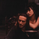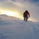# The chance of BTCUSD correction increases.

Short
BITSTAMP:BTCUSD   Bitcoin / U.S. Dollar5731 views
The chance of BTCUSD correction increases. In my analysis, I summarize the signals pointing in the direction of the correction and determine the size of the possible correction. 1. The fractal wave sequence is complete. (green square) The top of the second rising wave is 40441 usd. 2. This level is exactly equal to the symmetry of the fractal wave axis of the second wave (orange-yellow square). This is a symmetrical wave motion that is symmetrical to the W1 wave axis. CORRECTION SIZE: The size of the correction is obtained from the size of the first fractal correction. This is 27%. I hope I have understood so far. So we have two rising fractal pairs. Size of both 967% According to fractal rules, the percentage of corrections will be similar. That is why we expect a 27% reduction. The question arises as to why we give the size of the motion as a percentage? The answer is simple. Because the vertical scale on most trading platforms is a logarithmic scale. It follows that the magnitude of the correction in the two fractals is double, but the percentage is equal. In our case, this means that a corrective movement of up to 30614 usd can occur. I suggest investors to buy bitcoin in this correction (because the medium-term target price of 66,000 usd does not change) and Daytraders to think about taking a short position.Nice chart, would you not expect a further decline from 30,000, from an investors position there doesn't seem any value in purchasing BTC at these current prices. Maybe 18-20k could be more realisticDon_Knowles
@Don_Knowles, Yes. I agree with you. Through fractal calculations, I don’t see the 20,000 usd level yet. It is possible to adjust it in 1-2 days, taking into account additional incoming data. We'll see.